2020/09/21 10:54

一、概述

ConcurrentHashMap (以下简称C13Map) 是并发编程出场率最高的数据结构之一，大量的并发CASE背后都有C13Map的支持，同时也是JUC包中代码量最大的组件(6000多行)，自JDK8开始Oracle对其进行了大量优化工作。

二、HashMap基础知识

• 哈希表的长度永远都是2的幂次方，原因是hashcode%tableSize==hashcode&(tableSize-1)，也就是哈希槽位的确定可以用一次与运算来替代取余运算。
• 会对hashcode调用若干次扰动函数，将高16位与低16位做异或运算，因为高16位的随机性更强。
• 当表中的元素总数超过tableSize * 0.75时，哈希表会发生扩容操作，每次扩容的tableSize是原先的两倍。
• 下文提到的槽位(bucket)、哈希分桶、BIN均表示同一个概念，即哈希table上的某一列。
• 旧表在做搬运时i槽位的node可以根据其哈希值的第tableSize位的bit决定在新表上的槽位是i还是i+tableSize。
• 每个槽位上有可能会出现哈希冲突，在未达到某个阈值时它是一个链表结构，达到阈值后会升级到红黑树结构。
• HashMap本身并非为多线程环境设计，永远不要尝试在并发环境下直接使用HashMap，C13Map不存在这个安全问题。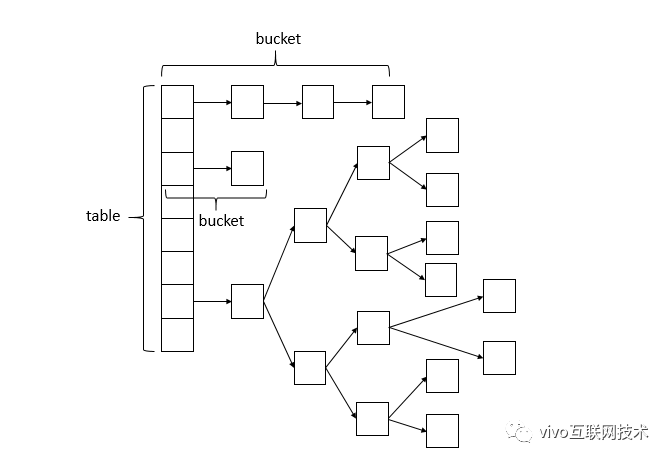三、C13Map的字段定义

C13Map的字段定义

//最大容量
private static final int MAXIMUM_CAPACITY = 1 << 30;

//默认初始容量
private static final int DEFAULT_CAPACITY = 16;

//数组的最大容量,防止抛出OOM
static final int MAX_ARRAY_SIZE = Integer.MAX_VALUE - 8;

//最大并行度，仅用于兼容JDK1.7以前版本
private static final int DEFAULT_CONCURRENCY_LEVEL = 16;

//扩容因子
private static final float LOAD_FACTOR = 0.75f;

//链表转红黑树的阈值
static final int TREEIFY_THRESHOLD = 8;

//红黑树退化阈值
static final int UNTREEIFY_THRESHOLD = 6;

//链表转红黑树的最小总量
static final int MIN_TREEIFY_CAPACITY = 64;

//扩容搬运时批量搬运的最小槽位数
private static final int MIN_TRANSFER_STRIDE = 16;

//当前待扩容table的邮戳位,通常是高16位
private static final int RESIZE_STAMP_BITS = 16;

//同时搬运的线程数自增的最大值
private static final int MAX_RESIZERS = (1 << (32 - RESIZE_STAMP_BITS)) - 1;

//搬运线程数的标识位，通常是低16位
private static final int RESIZE_STAMP_SHIFT = 32 - RESIZE_STAMP_BITS;

static final int MOVED     = -1; // 说明是forwardingNode
static final int TREEBIN   = -2; // 红黑树
static final int RESERVED  = -3; // 原子计算的占位Node
static final int HASH_BITS = 0x7fffffff; // 保证hashcode扰动计算结果为正数

//当前哈希表
transient volatile Node<K,V>[] table;

//下一个哈希表
private transient volatile Node<K,V>[] nextTable;

//计数的基准值
private transient volatile long baseCount;

//控制变量，不同场景有不同用途，参考下文
private transient volatile int sizeCtl;

//并发搬运过程中CAS获取区段的下限值
private transient volatile int transferIndex;

//计数cell初始化或者扩容时基于此字段使用自旋锁
private transient volatile int cellsBusy;

//加速多核CPU计数的cell数组
private transient volatile CounterCell[] counterCells;

四、安全操作Node<K,V>数组

static final <K,V> Node<K,V> tabAt(Node<K,V>[] tab, int i) {
return (Node<K,V>)U.getReferenceAcquire(tab, ((long)i << ASHIFT) + ABASE);
}
static final <K,V> boolean casTabAt(Node<K,V>[] tab, int i,
Node<K,V> c, Node<K,V> v) {
return U.compareAndSetReference(tab, ((long)i << ASHIFT) + ABASE, c, v);
}
static final <K,V> void setTabAt(Node<K,V>[] tab, int i, Node<K,V> v) {
U.putReferenceRelease(tab, ((long)i << ASHIFT) + ABASE, v);
}

五、读操作get为什么是线程安全的

C13Map的get方法

public V get(Object key) {
Node<K,V>[] tab; Node<K,V> e, p; int n, eh; K ek;
//执行扰动函数
if ((tab = table) != null && (n = tab.length) > 0 && (e = tabAt(tab, (n - 1) & h)) != null) {
if ((eh = e.hash) == h) {
if ((ek = e.key) == key || (ek != null && key.equals(ek)))
return e.val;
}
else if (eh < 0)
return (p = e.find(h, key)) != null ? p.val : null;
while ((e = e.next) != null) {
if (e.hash == h &&
((ek = e.key) == key || (ek != null && key.equals(ek))))
return e.val;
}
}
return null;
}

3、如果读取的bin是一个链表

（1）如果正在发生链表向红黑树的treeify工作，因为treeify本身并不破坏旧的链表bin的结构，只是在全部treeify完成后将头节点一次性替换为新创建的TreeBin，可以放心读取。

（2）如果正在发生resize且当前bin正在被transfer，因为transfer本身并不破坏旧的链表bin的结构，只是在全部transfer完成后将头节点一次性替换为ForwardingNode，可以放心读取。

• 如果是remove操作，存在遍历到某个Node时，正好有其它线程将其remove，导致其孤立于整个链表之外；但因为其next引用未发生变更，整个链表并没有断开，还是可以照常遍历链表直到tailNode。
• 如果是replace操作，链表的结构未变，只是某个Node的value发生了变化，没有安全问题。

4、如果读取的bin是一个红黑树

（1）如果正在发生红黑树向链表的untreeify操作，因为untreeify本身并不破坏旧的红黑树结构，只是在全部untreeify完成后将头节点一次性替换为新创建的普通Node，可以放心读取。

（2）如果正在发生resize且当前bin正在被transfer，因为transfer本身并不破坏旧的红黑树结构，只是在全部transfer完成后将头节点一次性替换为ForwardingNode，可以放心读取。

TreeBin中的读写锁实现

TreeNode<K,V> root;
volatile TreeNode<K,V> first;
volatile int lockState;
// values for lockState
static final int WRITER = 1; // set while holding write lock
static final int WAITER = 2; // set when waiting for write lock
static final int READER = 4; // increment value for setting read lock

private final void lockRoot() {
//如果一次性获取写锁失败，进入contendedLock循环体，循环获取写锁或者休眠等待
if (!U.compareAndSetInt(this, LOCKSTATE, 0, WRITER))
contendedLock(); // offload to separate method
}

private final void unlockRoot() {
lockState = 0;
}
//对红黑树加互斥锁,也就是写锁
private final void contendedLock() {
boolean waiting = false;
for (int s;;) {
//如果lockState除了第二位外其它位上都为0，表示红黑树当前既没有上读锁，又没有上写锁，仅有可能存在waiter，可以尝试直接获取写锁
if (((s = lockState) & ~WAITER) == 0) {
if (U.compareAndSetInt(this, LOCKSTATE, s, WRITER)) {
if (waiting)
waiter = null;
return;
}
}
//如果lockState第二位是0,表示当前没有线程在等待写锁
else if ((s & WAITER) == 0) {
//将lockState的第二位设置为1，相当于打上了waiter的标记，表示有线程在等待写锁
if (U.compareAndSetInt(this, LOCKSTATE, s, s | WAITER)) {
waiting = true;
}
}
//休眠当前线程
else if (waiting)
LockSupport.park(this);
}
}

//查找红黑树中的某个节点
final Node<K,V> find(int h, Object k) {
if (k != null) {
for (Node<K,V> e = first; e != null; ) {
int s; K ek;
//如果当前有waiter或者有写锁，走线性检索，因为红黑树虽然替代了链表，但其内部依然保留了链表的结构，虽然链表的查询性能一般，但根据先前的分析其读取的安全性有保证。
//发现有写锁改走线性检索，是为了避免等待写锁释放花去太久时间; 而发现有waiter改走线性检索，是为了避免读锁叠加的太多，导致写锁线程需要等待太长的时间; 本质上都是为了减少读写碰撞
//线性遍历的过程中，每遍历到下一个节点都做一次判断，一旦发现锁竞争的可能性减少就改走tree检索以提高性能
if (((s = lockState) & (WAITER|WRITER)) != 0) {
if (e.hash == h &&
((ek = e.key) == k || (ek != null && k.equals(ek))))
return e;
e = e.next;
}
//对红黑树加共享锁,也就是读锁,CAS一次性增加4，也就是增加的只是3~32位
else if (U.compareAndSetInt(this, LOCKSTATE, s,
TreeNode<K,V> r, p;
try {
p = ((r = root) == null ? null :
r.findTreeNode(h, k, null));
} finally {
//释放读锁，如果释放完毕且有waiter,则将其唤醒
(READER|WAITER) && (w = waiter) != null)
LockSupport.unpark(w);
}
return p;
}
}
}
return null;
}
//更新红黑树中的某个节点
final TreeNode<K,V> putTreeVal(int h, K k, V v) {
Class<?> kc = null;
boolean searched = false;
for (TreeNode<K,V> p = root;;) {
int dir, ph; K pk;
//...省略处理红黑树数据结构的代码若干
else {
//写操作前加互斥锁
lockRoot();
try {
root = balanceInsertion(root, x);
} finally {
//释放互斥锁
unlockRoot();
}
}
break;
}
}
assert checkInvariants(root);
return null;
}
}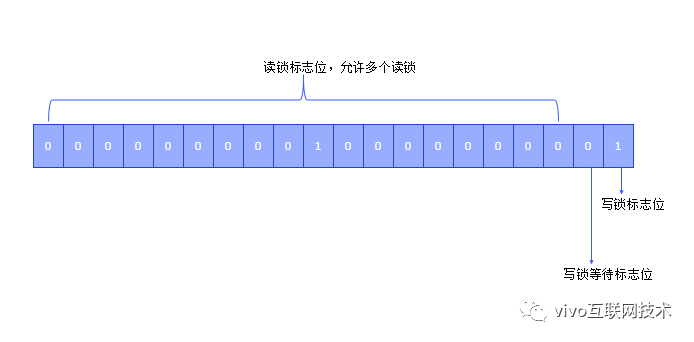TreeBin在find读操作检索时，在linearSearch(线性检索)和treeSearch(树检索)间做了折衷，前者性能差但并发安全，后者性能佳但要做并发控制，可能导致锁竞争；设计者使用线性检索来尽量避免读写碰撞导致的锁竞争，但评估到race condition已消失时，又立即趋向于改用树检索来提高性能，在安全和性能之间做到了极佳的平衡。具体的折衷策略请参考find方法及注释。

5、如果读取的bin是一个ForwardingNode

forwardingNode的find方法

static final class ForwardingNode<K,V> extends Node<K,V> {
final Node<K,V>[] nextTable;
ForwardingNode(Node<K,V>[] tab) {
super(MOVED, null, null);
this.nextTable = tab;
}

//递归检索哈希表链
Node<K,V> find(int h, Object k) {
// loop to avoid arbitrarily deep recursion on forwarding nodes
outer: for (Node<K,V>[] tab = nextTable;;) {
Node<K,V> e; int n;
if (k == null || tab == null || (n = tab.length) == 0 ||
(e = tabAt(tab, (n - 1) & h)) == null)
return null;
for (;;) {
int eh; K ek;
if ((eh = e.hash) == h &&
((ek = e.key) == k || (ek != null && k.equals(ek))))
return e;
if (eh < 0) {
if (e instanceof ForwardingNode) {
tab = ((ForwardingNode<K,V>)e).nextTable;
continue outer;
}
else
return e.find(h, k);
}
if ((e = e.next) == null)
return null;
}
}
}

}

ForwardingNode中保存了nextTable的引用，会转向下一个哈希表进行检索，但并不能保证nextTable就一定是currentTable，因为在高并发插入的情况下，极短时间内就可以导致哈希表的多次扩容，内存中极有可能驻留一条哈希表链，彼此以bin的头节点上的ForwardingNode相连，线程刚读取时拿到的是table1，遍历时却有可能经历了哈希表的链条。

eh<0有三种情况：

• 如果是ForwardingNode继续遍历下一个哈希表。
• 如果是TreeBin,调用其find方法进入TreeBin读写锁的保护区读取数据。
• 如果是ReserveNode，说明当前有compute计算中，整条bin还是一个空结构，直接返回null。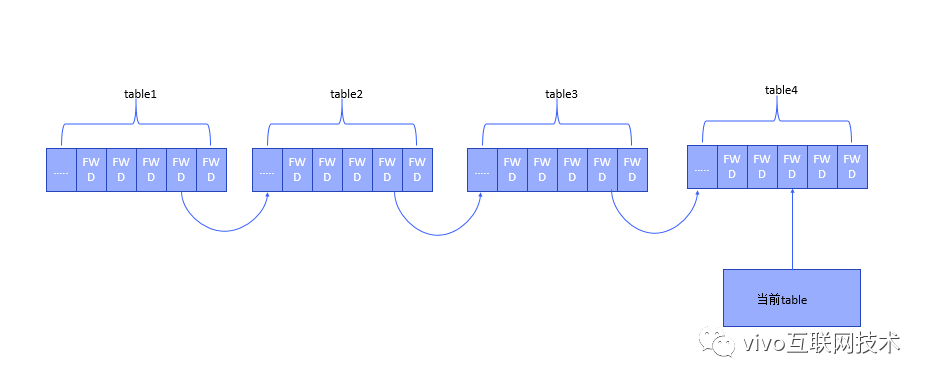6、如果读取的bin是一个ReserveNode

ReserveNode用于compute/computeIfAbsent原子计算的方法，在BIN的头节点为null且计算尚未完成时，先在bin的头节点打上一个ReserveNode的占位标记。

六、写操作putValue/replaceNode为什么是线程安全的

C13Map的putValue方法

Node<K,V>[] tab = table;  //将堆中的table变量赋给线程堆栈中的局部变量
Node f = tabAt(tab, i );
if(f==null){
//当前槽位没有头节点,直接CAS写入
if (casTabAt(tab, i, null, new Node<K,V>(hash, key, value)))
break;
}else if(f.hash == MOVED){
//加入协助搬运行列
helpTransfer(tab,f);
}
//不是forwardingNode
else if(f.hash != MOVED){
//先锁住I槽位上的头节点
synchronized (f) {
//再doubleCheck看此槽位上的头节点是否还是f
if (tabAt(tab, i) == f) {
...各种写操作
}
}
}

七、原子计算相关方法

1、几个方法的比较

（1）computeIfAbsent只会在判断到key不存在时才会插入，判空与插入是一个原子操作，提供的FunctionalInterface是一个二元的Function, 接受key参数，返回value结果；如果计算结果为null则不做插入。

（2）computeIfPresent只会在判读单到Key非空时才会做更新，判断非空与插入是一个原子操作，提供的FunctionalInterface是一个三元的BiFunction,接受key,value两个参数，返回新的value结果；如果新的value为null则删除key对应节点。

（3）compute则不加key是否存在的限制，提供的FunctionalInterface是一个三元的BiFunction,接受key,value两个参数，返回新的value结果；如果旧的value不存在则以null替代进行计算；如果新的value为null则保证key对应节点不会存在。

（4）merge不加key是否存在的限制，提供的FunctionalInterface是一个三元的BiFunction,接受oldValue, newVALUE两个参数，返回merge后的value；如果旧的value不存在，直接以newVALUE作为最终结果，存在则返回merge后的结果；如果最终结果为null，则保证key对应节点不会存在。

2、何时会使用ReserveNode占位

（1）为什么只有computeIfAbsent/compute方法使用占位符的方式

computeIfPresent只有在BIN结构非空的情况下才会展开原子计算，自然不存在需要ReserveNode占位的情况；锁住已有的头节点即可。

computeIfAbsent/compute方法在BIN结构为空时，需要展开Function或者BiFunction的运算，这个操作是外部引入的需要耗时多久无法准确评估；这种情况下如果采用先计算，再casTabAt(tab, i, null, r)的方式，如果有其它线程提前更新了这个BIN，那么就需要重新锁定新加入的头节点，并重复一次原子计算(C13Map无法帮你缓存上次计算的结果，因为计算的入参有可能会变化)，这个开销是比较大的。

（2）merge方法为何不需要占位

C13Map的compute方法

public V compute(K key,
BiFunction<? super K, ? super V, ? extends V> remappingFunction) {
if (key == null || remappingFunction == null)
throw new nullPointerException();
V val = null;
int delta = 0;
int binCount = 0;
for (Node<K, V>[] tab = table; ; ) {
Node<K, V> f;
int n, i, fh;
if (tab == null || (n = tab.length) == 0)
tab = initTable();
else if ((f = tabAt(tab, i = (n - 1) & h)) == null) {
//创建占位Node
Node<K, V> r = new ReservationNode<K, V>();
//先锁定该占位Node
synchronized (r) {
//将其设置到BIN的头节点
if (casTabAt(tab, i, null, r)) {
binCount = 1;
Node<K, V> node = null;
try {
//开始原子计算
if ((val = remappingFunction.apply(key, null)) != null) {
delta = 1;
node = new Node<K, V>(h, key, val, null);
}
} finally {
//设置计算后的最终节点
setTabAt(tab, i, node);
}
}
}
if (binCount != 0)
break;
} else if ((fh = f.hash) == MOVED)
tab = helpTransfer(tab, f);
else {
synchronized (f) {
if (tabAt(tab, i) == f) {
if (fh >= 0) {
//此处省略对普通链表的变更操作
} else if (f instanceof TreeBin) {
//此处省略对红黑树的变更操作
}
}
}

}
}
if (delta != 0)
return val;
}

3、如何保证原子性

computeIfAbsent/computeIfPresent中判空与计算是原子操作，根据上述分析主要是通过casTabAt(tab, i, null, r)原子操作，或者使用ReserveNode占位并锁定的方式，或者锁住bin的头节点的方式来实现的。

八、resize过程中的并发transfer

• tryPresize是putAll一次性插入一个集合前的自检，如果集合数目较大，则预先触发一次resize扩容和旧表向新表的transfer。
• helpTransfer是写操作过程中发现bin的头节点是ForwardingNode, 则调用helpTransfer加入协助搬运的行列。

1、开始transfer前的检查工作

Node<K, V>[] tab, nt;
int n, sc;
//当前的tableSize已经超过sizeCtl阈值，且小于最大值
while (s >= (long) (sc = sizeCtl) && (tab = table) != null &&
(n = tab.length) < MAXIMUM_CAPACITY) {
int rs = resizeStamp(n);
//已经在搬运中
if (sc < 0) {
if ((sc >>> RESIZE_STAMP_SHIFT) != rs || sc == rs + 1 ||
sc == rs + MAX_RESIZERS || (nt = nextTable) == null ||
transferIndex <= 0)
break;
//搬运线程数加一
if (U.compareAndSwapInt(this, SIZECTL, sc, sc + 1))
transfer(tab, nt);
} else if (U.compareAndSwapInt(this, SIZECTL, sc,
(rs << RESIZE_STAMP_SHIFT) + 2))
//尚未搬运，当前线程是本次resize工作的第一个线程，设置初始值为2，非常巧妙的设计
transfer(tab, null);
s = sumCount();
}

• 初始值是0表示哈希表尚未初始化
• 如果是-1表示正在初始化，只允许一个线程进入初始化代码块
• 在扩容过程中，sizeCtrl是一个负整数，其高16位是与当前的tableSize关联的邮戳resizeStamp，其低16位是当前从事搬运工作的线程数加1

2、并发搬运过程和退出机制

C13Map的transfer方法

private final void transfer(Node<K, V>[] tab, Node<K, V>[] nextTab) {
int n = tab.length, stride;
//一次搬运多少个槽位
if ((stride = (NCPU > 1) ? (n >>> 3) / NCPU : n) < MIN_TRANSFER_STRIDE)
stride = MIN_TRANSFER_STRIDE;
if (nextTab == null) {
try {
//首个搬运线程，负责初始化nextTable
Node<K, V>[] nt = (Node<K, V>[]) new Node<?, ?>[n << 1];
nextTab = nt;
} catch (Throwable ex) {
sizeCtl = Integer.MAX_VALUE;
return;
}
nextTable = nextTab;
//初始化当前搬运索引
transferIndex = n;
}
int nextn = nextTab.length;
//公共的forwardingNode
ForwardingNode<K, V> fwd = new ForwardingNode<K, V>(nextTab);
boolean finishing = false; // 保证提交nextTable之前已遍历旧表的所有槽位
for (int i = 0, bound = 0; ; ) {
Node<K, V> f;
int fh;
//循环CAS获取下一个搬运区段
int nextIndex, nextBound;
//搬运已结束，或者当前区段尚未完成，退出循环体;最后一次抄底扫描时，仅辅助做i减一的运算
if (--i >= bound || finishing)
else if ((nextIndex = transferIndex) <= 0) {
i = -1;
} else if (U.compareAndSwapInt
(this, TRANSFERINDEX, nextIndex,
nextBound = (nextIndex > stride ?
nextIndex - stride : 0))) {

bound = nextBound;
i = nextIndex - 1;
}
}
if (i < 0 || i >= n || i + n >= nextn) {
int sc;
if (finishing) {
nextTable = null;
table = nextTab;
sizeCtl = (n << 1) - (n >>> 1);
return;
}
if (U.compareAndSwapInt(this, SIZECTL, sc = sizeCtl, sc - 1)) {
//并非最后一个退出的线程
if ((sc - 2) != resizeStamp(n) << RESIZE_STAMP_SHIFT)
return;
//异常巧妙的设计，最后一个线程推出前将i回退到最高位，等于是强制做最后一次的全表扫描；程序直接执行后续的else if代码，看有没有哪个槽位漏掉了，或者说是否全部是forwardingNode标记；
//可以视为抄底逻辑，虽然检测到漏掉槽位的概率基本是0
i = n;
}
} else if ((f = tabAt(tab, i)) == null)
//空槽位直接打上forwardingNode标记，CAS失败下一次循环继续搬运该槽位，成功则进入下一个槽位
advance = casTabAt(tab, i, null, fwd);
else if ((fh = f.hash) == MOVED)
else {
//锁定头节点
synchronized (f) {
if (tabAt(tab, i) == f) {
Node<K, V> ln, hn;
if (fh >= 0) {
//......此处省略链表搬运代码:职责是将链表拆成两份,搬运到nextTable的i和i+n槽位
setTabAt(nextTab, i, ln);
setTabAt(nextTab, i + n, hn);
//设置旧表对应槽位的头节点为forwardingNode
setTabAt(tab, i, fwd);
} else if (f instanceof TreeBin) {
//......此处省略红黑树搬运代码:职责是将红黑树拆成两份,搬运到nextTable的i和i+n槽位，如果满足红黑树的退化条件，顺便将其退化为链表
setTabAt(nextTab, i, ln);
setTabAt(nextTab, i + n, hn);
//设置旧表对应槽位的头节点为forwardingNode
setTabAt(tab, i, fwd);
}
}
}
}
}
}

3、transfer过程中的读写安全性分析

（1）首先是transfer过程中是否有可能全局的哈希表table发生多次resize，或者说存在过期的风险？

（2）有并发的写操作时，是否存在安全风险？

nextTable的提交总是在所有的槽位都已经搬运完毕，插上ForwardingNode的标识之后的，因此只要新表已提交，旧表必定无法写入；这样就能够有效的避免数据写入旧表。

（3）有并发的读操作时，是否存在安全风险？

九、Traverser遍历器

C13Map的Traverser组件

static class Traverser<K,V> {
Node<K,V>[] tab;        // current table; updated if resized
Node<K,V> next;         // the next entry to use
TableStack<K,V> stack, spare; // to save/restore on ForwardingNodes
int index;              // index of bin to use next
int baseIndex;          // current index of initial table
int baseLimit;          // index bound for initial table
final int baseSize;     // initial table size

Traverser(Node<K,V>[] tab, int size, int index, int limit) {
this.tab = tab;
this.baseSize = size;
this.baseIndex = this.index = index;
this.baseLimit = limit;
this.next = null;
}

/**
* 返回下一个节点
*/
Node<K,V> e;
if ((e = next) != null)
e = e.next;
for (;;) {
Node<K,V>[] t; int i, n;  // 局部变量保证稳定性
if (e != null)
return next = e;
if (baseIndex >= baseLimit || (t = tab) == null ||
(n = t.length) <= (i = index) || i < 0)
return next = null;
if ((e = tabAt(t, i)) != null && e.hash < 0) {
if (e instanceof ForwardingNode) {
tab = ((ForwardingNode<K,V>)e).nextTable;
e = null;
pushState(t, i, n);
continue;
}
else if (e instanceof TreeBin)
e = ((TreeBin<K,V>)e).first;
else
e = null;
}
//当前如果有跳转堆栈直接回放
if (stack != null)
recoverState(n);
//没有跳转堆栈说明已经到initalTable
else if ((index = i + baseSize) >= n)
index = ++baseIndex; // visit upper slots if present
}
}

/**
* 遇到ForwardingNode时保存当前上下文
*/
private void pushState(Node<K,V>[] t, int i, int n) {
TableStack<K,V> s = spare;  // reuse if possible
if (s != null)
spare = s.next;
else
s = new TableStack<K,V>();
s.tab = t;
s.length = n;
s.index = i;
s.next = stack;
stack = s;
}

/**
* 弹出上下文
*
*/
private void recoverState(int n) {
TableStack<K,V> s; int len;
//如果当前有堆栈，且index已经到达右半段后溢出当前table,说明该回去了
//如果index还在左半段，则只辅助做index+=s.length操作
while ((s = stack) != null && (index += (len = s.length)) >= n) {
n = len;
index = s.index;
tab = s.tab;
s.tab = null;
TableStack<K,V> next = s.next;
s.next = spare; // save for reuse
stack = next;
spare = s;
}
//已经到initialTable,索引自增
if (s == null && (index += baseSize) >= n)
index = ++baseIndex;
}
}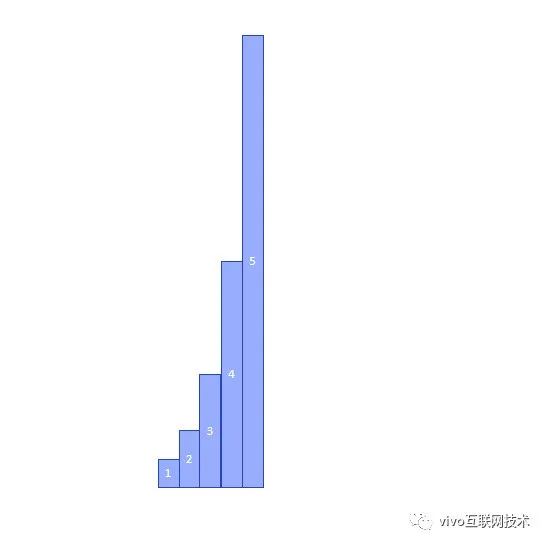traverser并不能保证一定遍历某张表的所有的槽位，但如果假设低阶表的某个槽位在最高阶表总是有相应的投影，比如table1的一个节点在table5中就会对应16个投影；

traverser能够保证一次遍历的所有槽位在最高阶表上的投影，可以布满整张最高阶表，而不会有任何遗漏。

十、并发计数

CounterCell[]机制并不理会新旧table的更迭，不管是操作的新表还是旧表，对于计数而言没有本质的差异，CounterCell[]只关注总量的增加或减少。

2、CounterCell[] 、cellBusy、baseCount的作用

CounterCell[]中存放2的指数幂个CounterCell，并发操作期间有可能会扩容，每次扩容都是原有size的两倍，一旦超过了CPU的核数即不再扩容，因为CPU的总数通常也是2的指数幂，所以其size往往等于CPU的核数CounterCell[]初始化、扩容、填充元素时，借助cellBusy其进行spinLock控制baseCount是基础数据。

private final void addCount(long x, int check) {
CounterCell[] cs; long b, s;
//初始时总是直接对baseCount计数，直到出现第一次失败，或者已经有现成的CounterCell[]数组可用
if ((cs = counterCells) != null ||
!U.compareAndSetLong(this, BASECOUNT, b = baseCount, s = b + x)) {
CounterCell c; long v; int m;
//是否存在竞态，为true时表示无竞态
boolean uncontended = true;
if (cs == null || (m = cs.length - 1) < 0 ||
//先生成随机数再对CounterCell[]数组size求余,也就是随机分配到其中某个槽位
(c = cs[ThreadLocalRandom.getProbe() & m]) == null ||
!(uncontended =
U.compareAndSetLong(c, CELLVALUE, v = c.value, v + x))) {
//该槽位尚未初始化或者CAS操作又出现竞态
return;
}
if (check <= 1)
return;
s = sumCount();
}
//检测元素总数是否超过sizeCtl阈值
if (check >= 0) {
Node<K,V>[] tab, nt; int n, sc;
while (s >= (long)(sc = sizeCtl) && (tab = table) != null &&
(n = tab.length) < MAXIMUM_CAPACITY) {
int rs = resizeStamp(n) << RESIZE_STAMP_SHIFT;
if (sc < 0) {
if (sc == rs + MAX_RESIZERS || sc == rs + 1 ||
(nt = nextTable) == null || transferIndex <= 0)
break;
if (U.compareAndSetInt(this, SIZECTL, sc, sc + 1))
transfer(tab, nt);
}
else if (U.compareAndSetInt(this, SIZECTL, sc, rs + 2))
transfer(tab, null);
s = sumCount();
}
}
}

private final void fullAddCount(long x, boolean wasUncontended) {
int h;
if ((h = ThreadLocalRandom.getProbe()) == 0) {
wasUncontended = true;
}
boolean collide = false;                // 是否有新的冲突
for (;;) {
CounterCell[] cs; CounterCell c; int n; long v;
if ((cs = counterCells) != null && (n = cs.length) > 0) {
if ((c = cs[(n - 1) & h]) == null) {
//随机匹配的槽位尚未有CounterCell元素则初始化之
if (cellsBusy == 0) {            // Try to attach new Cell
CounterCell r = new CounterCell(x); // Optimistic create
if (cellsBusy == 0 &&
U.compareAndSetInt(this, CELLSBUSY, 0, 1)) {
boolean created = false;
try {               // Recheck under lock
CounterCell[] rs; int m, j;
if ((rs = counterCells) != null &&
(m = rs.length) > 0 &&
rs[j = (m - 1) & h] == null) {
rs[j] = r;
created = true;
}
} finally {
cellsBusy = 0;
}
if (created)
break;
continue;           // Slot is now non-empty
}
}
collide = false;
}
else if (!wasUncontended)
else if (U.compareAndSetLong(c, CELLVALUE, v = c.value, v + x))
break;
else if (counterCells != cs || n >= NCPU)
collide = false;            // 超过CPU的最大核数，或者检测到counterCells已扩容，都将冲突状态置为无
else if (!collide)
collide = true;             // 以上的若干条件都不满足，可以判定必定有冲突，再生成一个随机数试探一下
else if (cellsBusy == 0 &&
U.compareAndSetInt(this, CELLSBUSY, 0, 1)) {
try {
if (counterCells == cs)   //对counterCells进行doubleCheck
counterCells = Arrays.copyOf(cs, n << 1);   //扩容，容量翻倍
} finally {
cellsBusy = 0;
}
collide = false;
continue;                   // 对性的counterCell[]进行重试CAS操作
}
}
else if (cellsBusy == 0 && counterCells == cs &&
U.compareAndSetInt(this, CELLSBUSY, 0, 1)) {
//第一次初始化工作，初始的数组大小为2
boolean init = false;
try {                           // Initialize table
if (counterCells == cs) {
CounterCell[] rs = new CounterCell;
rs[h & 1] = new CounterCell(x);
counterCells = rs;
init = true;
}
} finally {
cellsBusy = 0;
}
if (init)
break;
}
//初始化过程中其它线程的抄底方案
else if (U.compareAndSetLong(this, BASECOUNT, v = baseCount, v + x))
break;
}
}

CounterCell[]的整体方案相对独立，与C13Map的关系并不大，可以视为一种成熟的高性能技术方案在各个场景使用。

十一、与stream类似的bulk操作支持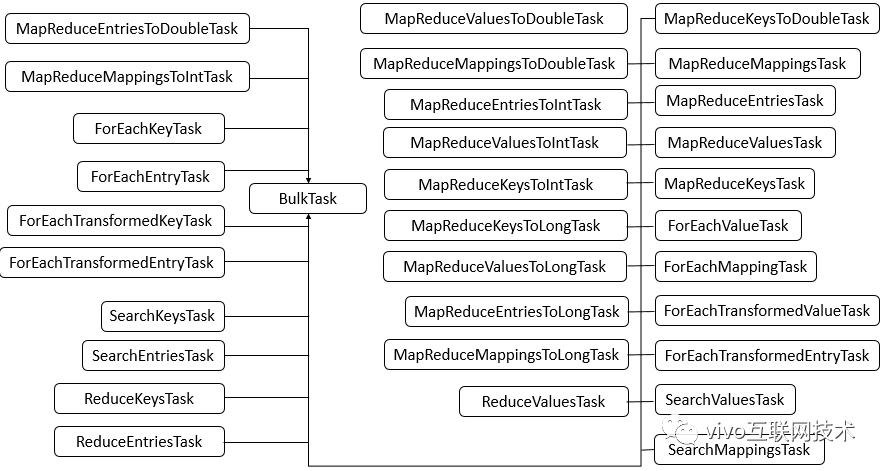2、几种有代表性的批量方法

C13Map的批量任务

//将所有的entry按照transformer函数进行二元计算，再对所有生成的结果执行action一元函数
public <U> void forEach(long parallelismThreshold,
BiFunction<? super K, ? super V, ? extends U> transformer,
Consumer<? super U> action);

//对所有的entry执行searchFunction二元计算，一旦发现任意一个计算结果不为null，即全盘返回
public <U> U search(long parallelismThreshold,
BiFunction<? super K, ? super V, ? extends U> searchFunction);

//对所有的entry执行transformer二元计算，再对所有的结果执行reducer收敛函数
public <U> U reduce(long parallelismThreshold,
BiFunction<? super K, ? super V, ? extends U> transformer,
BiFunction<? super U, ? super U, ? extends U> reducer)

//对所有的entry中的value执行transformer二元计算，再对所有的结果执行reducer收敛函数
public <U> U reduceValues(long parallelismThreshold,
Function<? super V, ? extends U> transformer,
BiFunction<? super U, ? super U, ? extends U> reducer)

final BiFunction<? super K, ? super V, ? extends U> transformer;
final BiFunction<? super U, ? super U, ? extends U> reducer;
U result;
(BulkTask<K,V,?> p, int b, int i, int f, Node<K,V>[] t,
BiFunction<? super K, ? super V, ? extends U> transformer,
BiFunction<? super U, ? super U, ? extends U> reducer) {
super(p, b, i, f, t); this.nextRight = nextRight;
this.transformer = transformer;
this.reducer = reducer;
}
public final U getRawResult() { return result; }
public final void compute() {
final BiFunction<? super K, ? super V, ? extends U> transformer;
final BiFunction<? super U, ? super U, ? extends U> reducer;
if ((transformer = this.transformer) != null &&
(reducer = this.reducer) != null) {
for (int i = baseIndex, f, h; batch > 0 &&
(h = ((f = baseLimit) + i) >>> 1) > i;) {
//裂变出新的fork-join任务
(this, batch >>>= 1, baseLimit = h, f, tab,
rights, transformer, reducer)).fork();
}
U r = null;
//遍历本batch元素
for (Node<K,V> p; (p = advance()) != null; ) {
U u;
//对本batch做reduce收敛操作
if ((u = transformer.apply(p.key, p.val)) != null)
r = (r == null) ? u : reducer.apply(r, u);
}
//对自己和自己fork出的子任务做reducer收敛操作
result = r;
CountedCompleter<?> c;
for (c = firstComplete(); c != null; c = c.nextComplete()) {
@SuppressWarnings("unchecked")
s = t.rights;
while (s != null) {
U tr, sr;
if ((sr = s.result) != null)
t.result = (((tr = t.result) == null) ? sr :
reducer.apply(tr, sr));
s = t.rights = s.nextRight;
}
}
}
}
}

十二、小结0
6 收藏

作者的其它热门文章0 评论
6 收藏
0